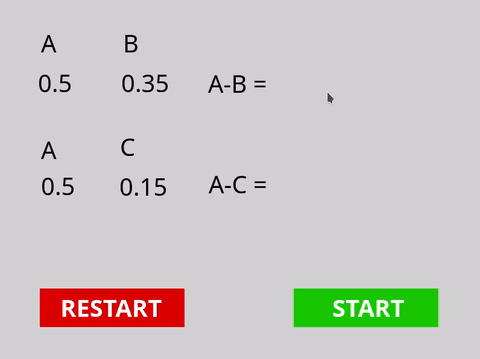# Subtraction bug?

I made a simple subtraction operation like this:

A=0.5
B=0.35
A-B=0.1500000000002 (wrong)
this should be 0.15

For comparison, I made a similar operation:

A=0.5
C=0.15
A-C=0.35 (right)

Is this a bug? Hope this gets fixed soon.GDevelop version : 5.0.138

tl;dr That’s due to how computers stores non-integer numbers (floats) and do math on them, and this cannot be fixed.

oh. maybe if I SubStr 0,150000000… to 4 letter (0,15) it will be correct. but I’m not sure.

If you know you only want 2 decimals, you could always use the roundTo() expression, it lets you round to a specific decimal point, so it’d be roundTo(yourmathhere,2).

Yes maybe roundTo or SubStr. I’m affraid if roundTo I will get 0,2.
So I try to multiply it with a large number , like 1000000000.
then do the subtraction to avoid the error.
(0.5x1000000000)-(0.35x1000000000)
then divide the result with 1000000000 again.
Maybe it work.

Can you also multiply by 100, use the floor function and divide by 100?
Or something using the floor function.?

Might be worth looking into the toFixed? There should be a handy extension for is.